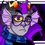# Exponentiation Question

What is $\displaystyle\lim_{n\rightarrow\infty}\dfrac{A}{B},$ where $A=n^{(n-1)^{(n-2)^{\ldots^{3^2}}}}$ and $B=2^{3^{4^{\ldots^{(n-1)^n}}}}$? I suspect it is $0,$ but I don't have any idea how I would go about proving this. A little help?Note by Trevor B.
5 years, 11 months ago

This discussion board is a place to discuss our Daily Challenges and the math and science related to those challenges. Explanations are more than just a solution — they should explain the steps and thinking strategies that you used to obtain the solution. Comments should further the discussion of math and science.

When posting on Brilliant:

• Use the emojis to react to an explanation, whether you're congratulating a job well done , or just really confused .
• Ask specific questions about the challenge or the steps in somebody's explanation. Well-posed questions can add a lot to the discussion, but posting "I don't understand!" doesn't help anyone.
• Try to contribute something new to the discussion, whether it is an extension, generalization or other idea related to the challenge.
• Stay on topic — we're all here to learn more about math and science, not to hear about your favorite get-rich-quick scheme or current world events.

MarkdownAppears as
*italics* or _italics_ italics
**bold** or __bold__ bold
- bulleted- list
• bulleted
• list
1. numbered2. list
1. numbered
2. list
Note: you must add a full line of space before and after lists for them to show up correctly
paragraph 1paragraph 2

paragraph 1

paragraph 2

[example link](https://brilliant.org)example link
> This is a quote
This is a quote
    # I indented these lines
# 4 spaces, and now they show
# up as a code block.

print "hello world"
# I indented these lines
# 4 spaces, and now they show
# up as a code block.

print "hello world"
MathAppears as
Remember to wrap math in $$ ... $$ or $ ... $ to ensure proper formatting.
2 \times 3 $2 \times 3$
2^{34} $2^{34}$
a_{i-1} $a_{i-1}$
\frac{2}{3} $\frac{2}{3}$
\sqrt{2} $\sqrt{2}$
\sum_{i=1}^3 $\sum_{i=1}^3$
\sin \theta $\sin \theta$
\boxed{123} $\boxed{123}$

## Comments

Sort by:

Top Newest

suppose the value of pie =0.31825. calculate the area of circle of radius= 5 equal to 78.55 . please share the answer ok.

- 5 years, 10 months ago

Log in to reply

I have a question, though. If the B grows faster, than the equation would near zero. If A grows faster, the equation would near infinity... right?

Am I missing something right now?

- 5 years, 10 months ago

Log in to reply

Wait, I think im pretty close.

- 5 years, 10 months ago

Log in to reply

The way I look at it, the limit of $\frac{A}{B}$ would be in the form $\frac{\inf}{\inf}$,

- 5 years, 11 months ago

Log in to reply

Certainly, but the question would be which sequence (see Calvin Lin's comment) grows faster.

- 5 years, 10 months ago

Log in to reply

Oh, ok, thanks

- 5 years, 10 months ago

Log in to reply

Those terms should be defined as $A_n$ and $B_n$.

Hint: How does $A_n$ and $A_{n-1}$ relate? What about $B_n$ and $B _{n-1}$?

Which test does that suggest we apply?

Staff - 5 years, 11 months ago

Log in to reply

While it is obvious that $A_n=n^{A_{n-1}},$ I can't come up with a mathematical relartion for $B_{n-1}$ and $B_n,$ since $B_n\neq B_{n-1}^n.$

- 5 years, 10 months ago

Log in to reply

×

Problem Loading...

Note Loading...

Set Loading...